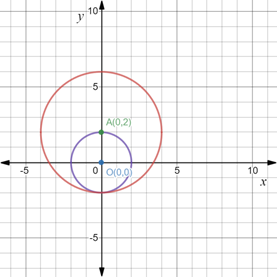# The area of the region that lies outside the circle x 2 + y 2 = 4 but inside the circle x 2 + y 2 − 4 y − 12 = 0 .### Precalculus: Mathematics for Calcu...

6th Edition
Stewart + 5 others
Publisher: Cengage Learning
ISBN: 9780840068071### Precalculus: Mathematics for Calcu...

6th Edition
Stewart + 5 others
Publisher: Cengage Learning
ISBN: 9780840068071

#### Solutions

Chapter 1.8, Problem 111E
To determine

## To calculate: The area of the region that lies outside the circle x2+y2=4 but inside the circle x2+y2−4y−12=0 .

Expert Solution

The area of the region is 12π sq. units .

### Explanation of Solution

Given information:

Equations of two circles x2+y2=4 and x2+y24y12=0 .

Formula used:

Standard equation of a circle passing through center (h,k) and having radius r is written as,

(xh)2+(yk)2=r2

Area of a circle with radius r units is product of pi (π) and square of its radius, which is mathematically expressed as,

Area=πr2

Calculation:

Consider the provided equations of x2+y2=4 and x2+y24y12=0 .

Recall the standard equation of a circle passing through center (h,k) and having radius r is written as,

(xh)2+(yk)2=r2

Comparing x2+y2=4 from the standard equation, we get center of the circle as (0,0) and its radius 2 units .

Now, convert x2+y24y12=0 into standard equation through the method of completing square by shifting 12 on right hand side and then adding 4 on both sides as,

x2+y24y=12x2+(y24y+4)=12+4x2+(y2)2=16(x0)2+(y2)2=42

Comparing x2+y24y12=0 from the standard equation, we get center of the circle as (0,2) and its radius 4 units .

Now, plot both the circle on the coordinate plane as,Area of the region that lies outside the circle x2+y2=4 but inside the circle x2+y24y12=0 will be calculated when area of smaller circle is subtracted from the area of bigger circle.

Recall area of a circle with radius r units is product of pi (π) and square of its radius, which is mathematically expressed as,

Area=πr2

So, area of bigger circle with radius 4 units is calculated as,

Abig=πr2=π(4)2=16π sq. units

Area of smaller circle with radius 2 units is calculated as,

Asmall=πr2=π(2)2=4π sq. units

Now, area of the region that lies outside the circle x2+y2=4 but inside the circle x2+y24y12=0 will be calculated as,

Aregion=AbigAsmall=(16π4π) sq. units=12π sq. units

Thus, the area of the region is 12π sq. units .

### Have a homework question?

Subscribe to bartleby learn! Ask subject matter experts 30 homework questions each month. Plus, you’ll have access to millions of step-by-step textbook answers!